Arrays Recall arrays char foo80 An array of

• Slides: 24Arrays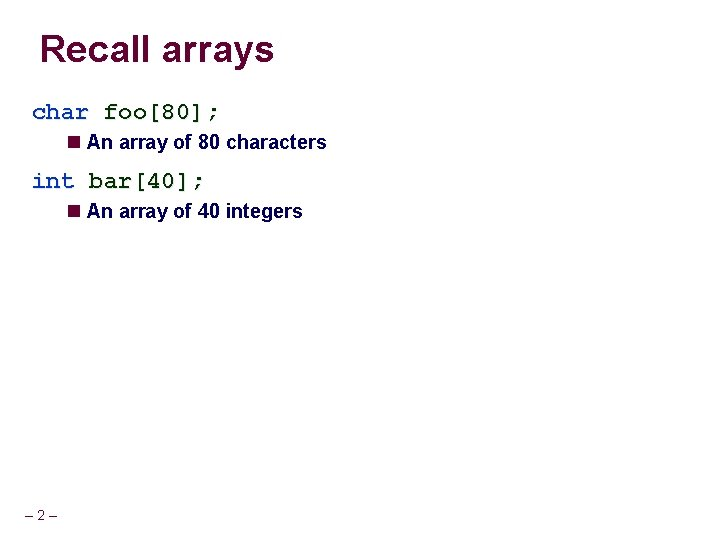Recall arrays char foo; An array of 80 characters int bar; An array of 40 integers – 2 –Array allocation Basic Principle T A[L]; A is an array of data type T and length L Contiguously allocated region of (L * sizeof(T)) bytes char string; x x + 12 int val; x long a; char *p; double a; x – 3 – x + 8 x + 4 x + 8 x + 16 x + 12 x + 16 x + 24 x + 20 x + 32Pointers and arrays closely related Name of array can be referenced as if it were a pointer long a; long *lptr; lptr = a; – 4 – /* a is array of 5 long /* lptr is a pointer to long /* set lptr to point to a */ */ */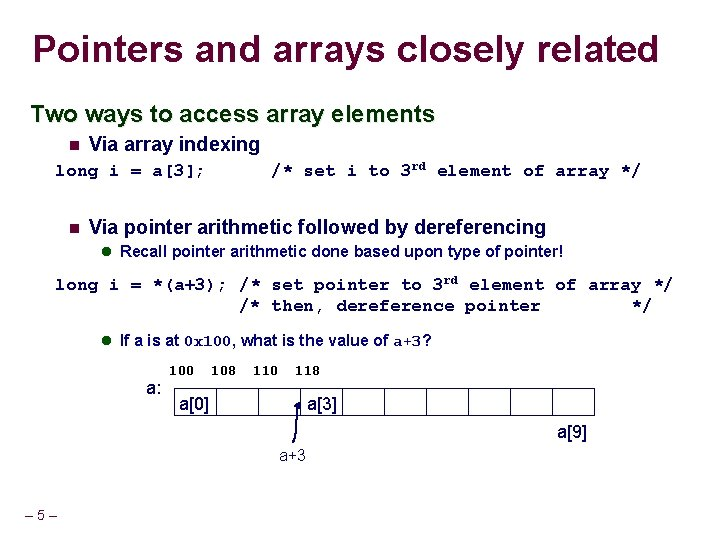Pointers and arrays closely related Two ways to access array elements Via array indexing /* set i to 3 rd element of array */ long i = a; Via pointer arithmetic followed by dereferencing Recall pointer arithmetic done based upon type of pointer! long i = *(a+3); /* set pointer to 3 rd element of array */ /* then, dereference pointer */ If a is at 0 x 100, what is the value of a+3? a: 100 108 110 118 a a a a+3 – 5 –Pointer arithmetic with arrays As a result of contiguous allocation Elements accessed by scaling the index by the size of the datum and adding to the start address Done via scaled index memory mode char long float – 6 – A; *B; C; D Array Element Size Total Size Start Address Element i A 1 12 x. A + i B 8 64 x. B + 8 i C 8 48 x. C + 8 i D 4 20 x. D + 4 iArray access examples val is an array at address x int val; 1 x 5 x + 4 2 x + 8 Reference Type Value val int 3 val int int int val+3 &val val *(val+1) val + i – 7 – * * x x+12 x+8 ? 5 x+4 i 1 3 x + 12 x + 16 x + 20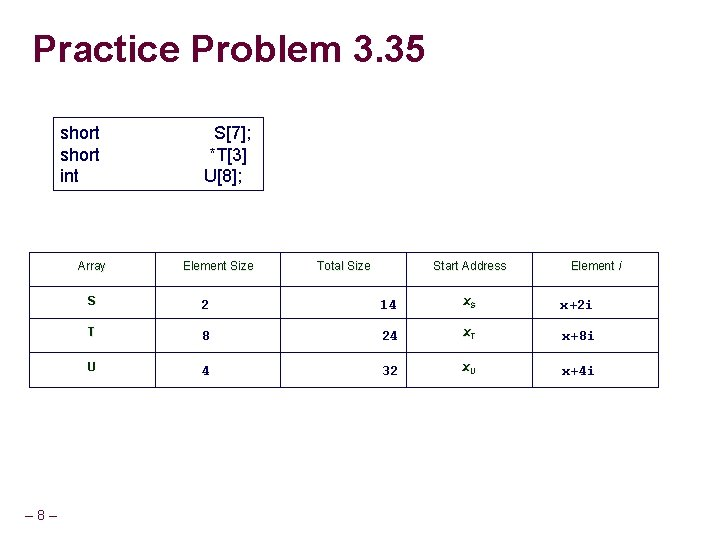Practice Problem 3. 35 – 8 – short int S; *T U; Array Element Size Total Size Start Address Element i S 2 14 x. S x+2 i T 8 24 x. T x+8 i U 4 32 x. U x+4 i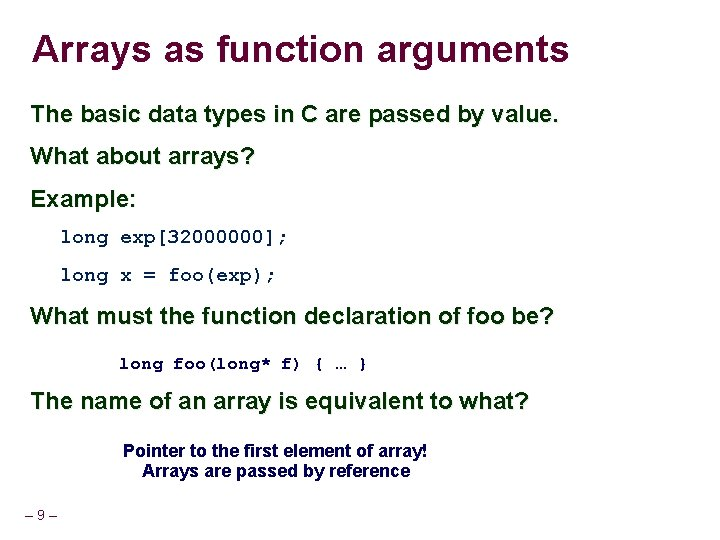Arrays as function arguments The basic data types in C are passed by value. What about arrays? Example: long exp; long x = foo(exp); What must the function declaration of foo be? long foo(long* f) { … } The name of an array is equivalent to what? Pointer to the first element of array! Arrays are passed by reference – 9 –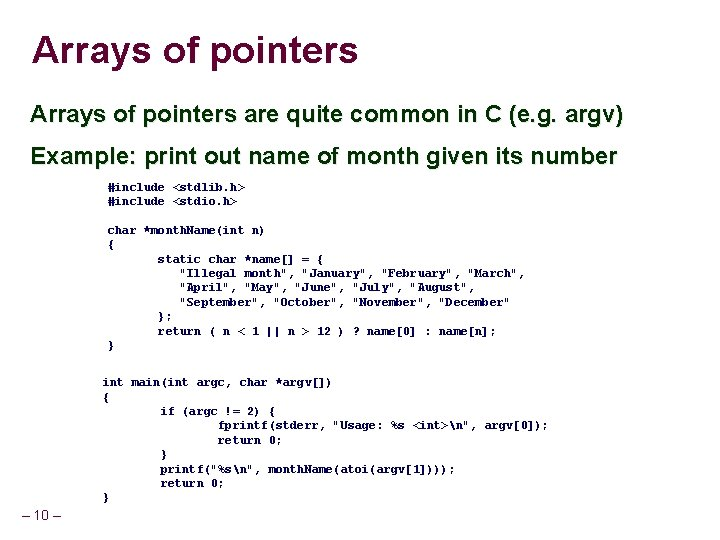Arrays of pointers are quite common in C (e. g. argv) Example: print out name of month given its number #include <stdlib. h> #include <stdio. h> char *month. Name(int n) { static char *name[] = { "Illegal month", "January", "February", "March", "April", "May", "June", "July", "August", "September", "October", "November", "December" }; return ( n < 1 || n > 12 ) ? name : name[n]; } int main(int argc, char *argv[]) { if (argc != 2) { fprintf(stderr, "Usage: %s <int>n", argv); return 0; } printf("%sn", month. Name(atoi(argv))); return 0; } – 10 –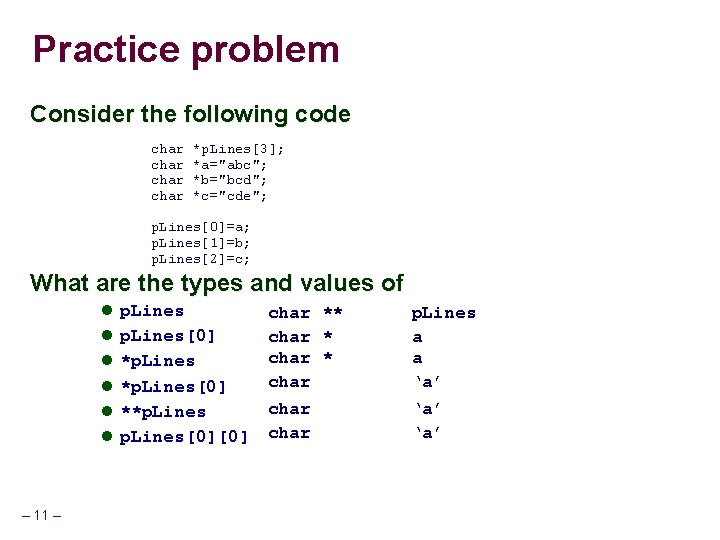Practice problem Consider the following code char *p. Lines; *a="abc"; *b="bcd"; *c="cde"; p. Lines=a; p. Lines=b; p. Lines=c; What are the types and values of p. Lines *p. Lines **p. Lines p. Lines – 11 – char ** char char p. Lines a a ‘a’ ‘a’Arrays in assembly Arrays typically have very regular access patterns – 12 – Optimizing compilers are very good at optimizing array indexing code As a result, output may not look at all like the inputArray access examples Pointer arithmetic int E; %rax == result %rdx == start address of E %rcx == index i Expression Type Value Assembly Code E int * XE movq %rdx, %rax E int M[XE] movl (%rdx), %eax E[i] int M[XE + 4 i ] movl (%rdx, %rcx, 4), %eax &E int * XE + 8 leaq 8(%rdx), %rax E+i-1 int * XE + 4 i - 4 leaq -4(%rdx, %rcx, 4), %rax *(&E[i]+i) int M[XE + 4 i ] movl (%rdx, %rcx, 8), %eax – 13 –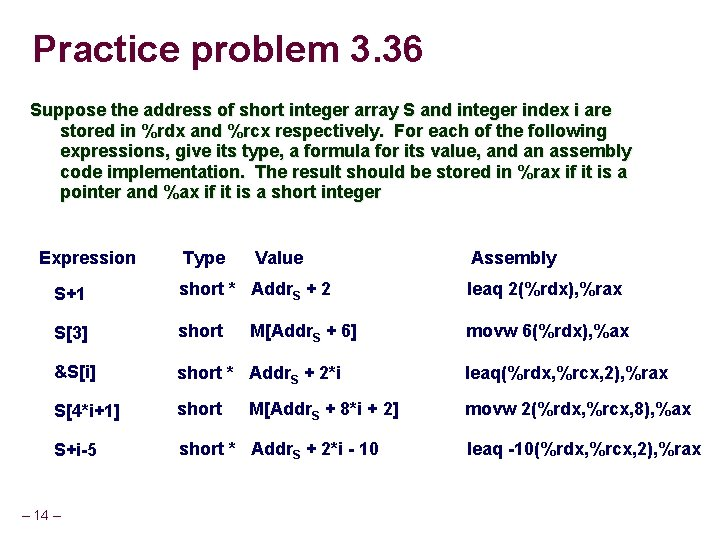Practice problem 3. 36 Suppose the address of short integer array S and integer index i are stored in %rdx and %rcx respectively. For each of the following expressions, give its type, a formula for its value, and an assembly code implementation. The result should be stored in %rax if it is a pointer and %ax if it is a short integer Expression Type Value Assembly S+1 short * Addr. S + 2 leaq 2(%rdx), %rax S short movw 6(%rdx), %ax &S[i] short * Addr. S + 2*i leaq(%rdx, %rcx, 2), %rax S[4*i+1] short movw 2(%rdx, %rcx, 8), %ax S+i-5 short * Addr. S + 2*i - 10 – 14 – M[Addr. S + 6] M[Addr. S + 8*i + 2] leaq -10(%rdx, %rcx, 2), %raxMulti-Dimensional Arrays C allows for multi-dimensional arrays int x[R][C]; x is an R x C matrix R rows, C elements/columns per row The dimensions of an array must be declared constants i. e. R and C, must be #define constants Compiler must be able to generate proper indexing code Can also have higher dimensions: x[A][B][C] where A, B, and C are constants Multidimensional arrays in C are stored in “row major” order Data grouped by rows All elements of a given row are stored contiguously A[*] = in contiguous memory followed by A[*] The last dimension is the one that varies the fastest with linear access through memory – 15 – Important to know for performance!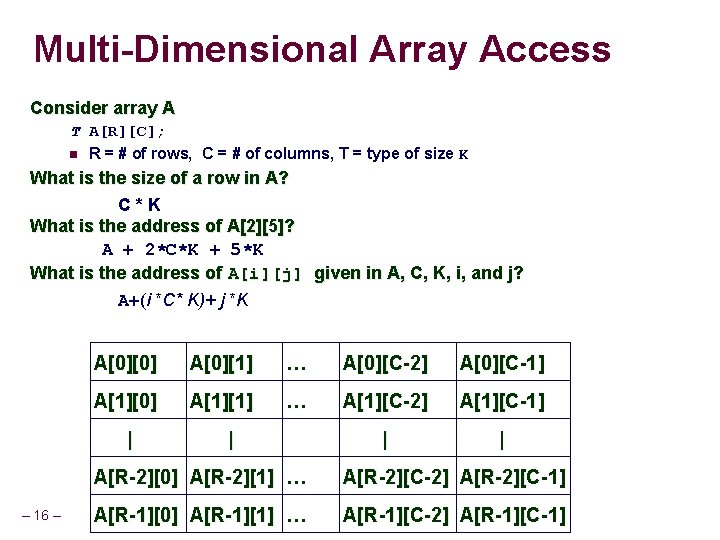Multi-Dimensional Array Access Consider array A T A[R][C]; R = # of rows, C = # of columns, T = type of size K What is the size of a row in A? C*K What is the address of A? A + 2*C*K + 5*K What is the address of A[i][j] given in A, C, K, i, and j? A+(i *C* K)+ j *K A A … A[C-2] A[C-1] A A … A[C-2] A[C-1] | | | – 16 – | A[R-2] A[R-2] … A[R-2][C-2] A[R-2][C-1] A[R-1] A[R-1] … A[R-1][C-2] A[R-1][C-1]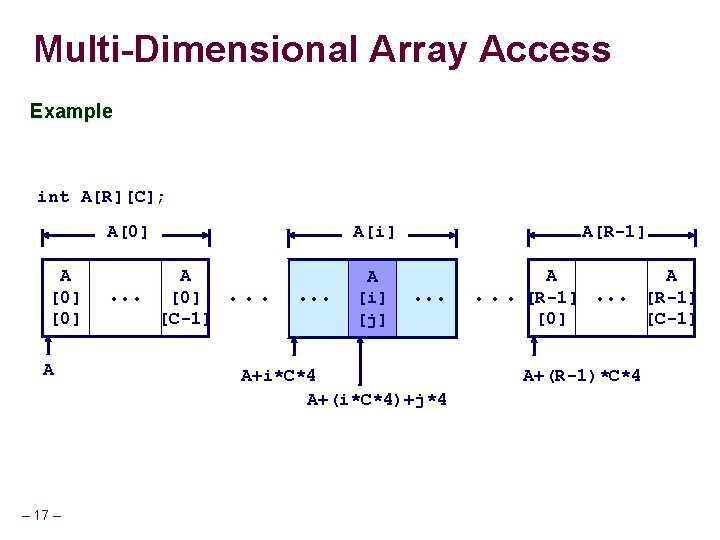Multi-Dimensional Array Access Example int A[R][C]; A A – 17 – • • • A[R-1] A[i] A  • • • [C-1] • • • A [i] [j] • • • A+i*C*4 A+(i*C*4)+j*4 A • • • [R-1]  • • • A+(R-1)*C*4 A [R-1] [C-1]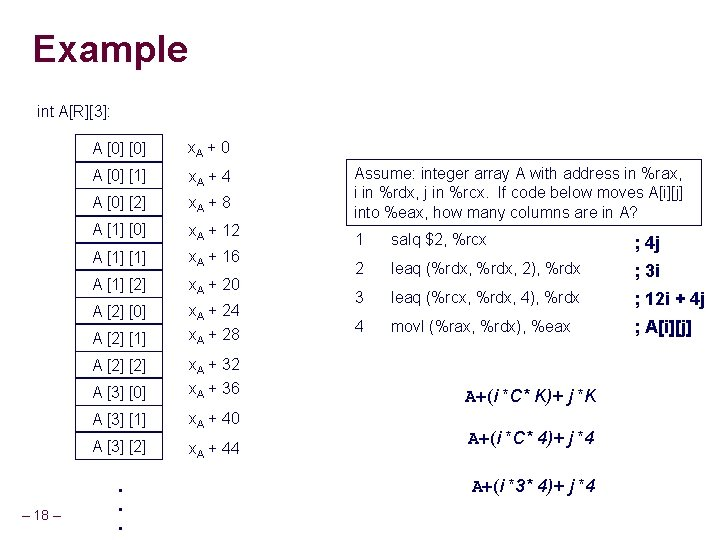Example int A[R]: A  x. A + 0 A   x. A + 4 A   x. A + 8 A   Assume: integer array A with address in %rax, i in %rdx, j in %rcx. If code below moves A[i][j] into %eax, how many columns are in A? x. A + 12 A  x. A + 16 1 salq \$2, %rcx ; 4 j A   x. A + 20 2 leaq (%rdx, 2), %rdx ; 3 i A   x. A + 24 x. A + 28 3 leaq (%rcx, %rdx, 4), %rdx ; 12 i + 4 j 4 movl (%rax, %rdx), %eax ; A[i][j] A   A   x. A + 32 x. A + 36 A   x. A + 40 A   x. A + 44 A  – 18 – . . . A+(i *C* K)+ j *K A+(i *C* 4)+ j *4 A+(i *3* 4)+ j *4Practice problem 3. 37 Assume M and N are #define constants. Given the following, what are their values? long P[M][N]; long Q[N][M]; long sum_element(long i, long j){ return (P[i][j] + Q[j][i]); } /* i in %rdi, j in %rsi */ sum_element: leaq 0(, %rdi, 8), %rdx subq %rdi, %rdx addq %rsi, %rdx leaq (%rsi, 4), %rax addq %rax, %rdi movq Q(, %rdi, 8), %rax addq P(, %rdx, 8), %rax – 19 – ; ; ; ; rdx rdx rax rdi rax = 8 i = 7 i + j = 5 j + i = M[Q+8*(5 j+i)] += M[P+8*(7 i+j)] Columns in Q => M=5 Columns in P => N=7Something to watch out for int A; // A has 12 rows of 13 ints each Will the C compiler permit us to do this? int x = A; What will happen? Indexing done assuming a 12 x 13 array A + (i*C + j) * K = A + (13*i + 26) * 4 = A + (13*(i+2) + 0) * 4 Same as A What about this? int x = A; C does not check array bounds – 20 – Contrast this to managed languages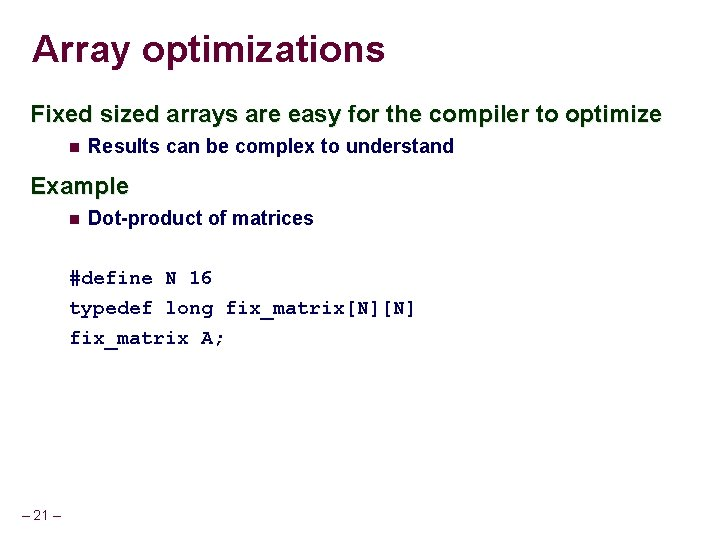Array optimizations Fixed sized arrays are easy for the compiler to optimize Results can be complex to understand Example Dot-product of matrices #define N 16 typedef long fix_matrix[N][N] fix_matrix A; – 21 –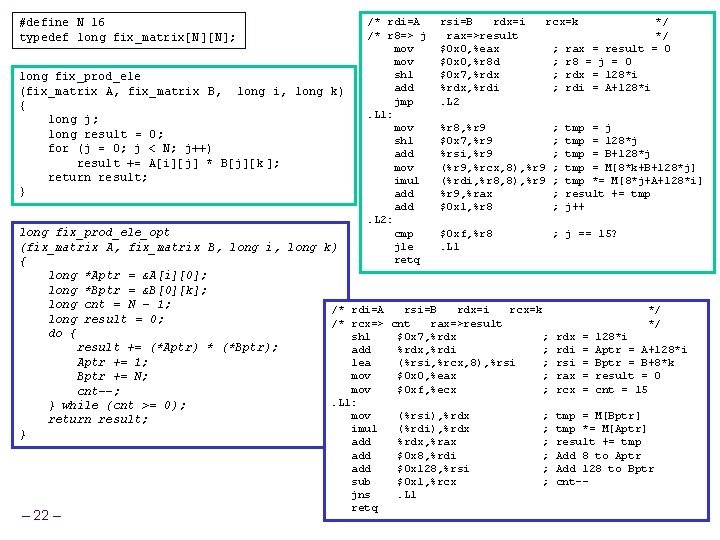#define N 16 typedef long fix_matrix[N][N]; long fix_prod_ele (fix_matrix A, fix_matrix B, long i, long k) { long j; long result = 0; for (j = 0; j < N; j++) result += A[i][j] * B[j][k ]; return result; } /* rdi=A /* r 8=> j mov shl add jmp. L 1: mov shl add mov imul add. L 2: cmp jle retq rsi=B rdx=i rax=>result \$0 x 0, %eax \$0 x 0, %r 8 d \$0 x 7, %rdx, %rdi. L 2 rcx=k %r 8, %r 9 \$0 x 7, %r 9 %rsi, %r 9 (%r 9, %rcx, 8), %r 9 (%rdi, %r 8, 8), %r 9, %rax \$0 x 1, %r 8 ; ; */ */ rax = result = 0 r 8 = j = 0 rdx = 128*i rdi = A+128*i ; ; ; ; tmp = j tmp = 128*j tmp = B+128*j tmp = M[8*k+B+128*j] tmp *= M[8*j+A+128*i] result += tmp j++ long fix_prod_ele_opt \$0 xf, %r 8 ; j == 15? . L 1 (fix_matrix A, fix_matrix B, long i, long k) { long *Aptr = &A[i]; long *Bptr = &B[k]; long cnt = N - 1; /* rdi=A rsi=B rdx=i rcx=k */ long result = 0; /* rcx=> cnt rax=>result */ do { shl \$0 x 7, %rdx ; rdx = 128*i result += (*Aptr) * (*Bptr); add %rdx, %rdi ; rdi = Aptr = A+128*i Aptr += 1; lea (%rsi, %rcx, 8), %rsi ; rsi = Bptr = B+8*k mov \$0 x 0, %eax ; rax = result = 0 Bptr += N; mov \$0 xf, %ecx ; rcx = cnt = 15 cnt--; . L 1: } while (cnt >= 0); mov (%rsi), %rdx ; tmp = M[Bptr] return result; imul (%rdi), %rdx ; tmp *= M[Aptr] } – 22 – add add sub jns retq %rdx, %rax \$0 x 8, %rdi \$0 x 128, %rsi \$0 x 1, %rcx. L 1 ; ; result += tmp Add 8 to Aptr Add 128 to Bptr cnt--Practice problem 3. 38 #define N 16 typedef long fix_matrix[N][N]; void fix_set_diag(fix_matrix A, long val) { long i; for (i=0; i<N; i++) A[i][i] = val; } mov. L 1: mov add cmp jne retq \$0 x 0, %eax %rsi, (%rdi, %rax, 8) \$17, %rax \$110, %rax. L 1 Note: Book uses int matrix, we use long Create a C code program fix_set_diag_opt that uses optimizations similar to those in the assembly code, in the same style as the previous slide void fix_set_diag_opt(fix_matrix long *Aptr = &A; /* long i = 0; /* long iend = N*(N+1); /* do { Aptr[i] = val; /* i += (N+1); /* } while (i != iend); /* } – 23 – A, long val) { Use Aptr to index into matrix */ Offset into Aptr for next element to set */ Stopping condition */ Index into memory i long ints */ Go down a row and forward one column */ Repeat until at top of matrix */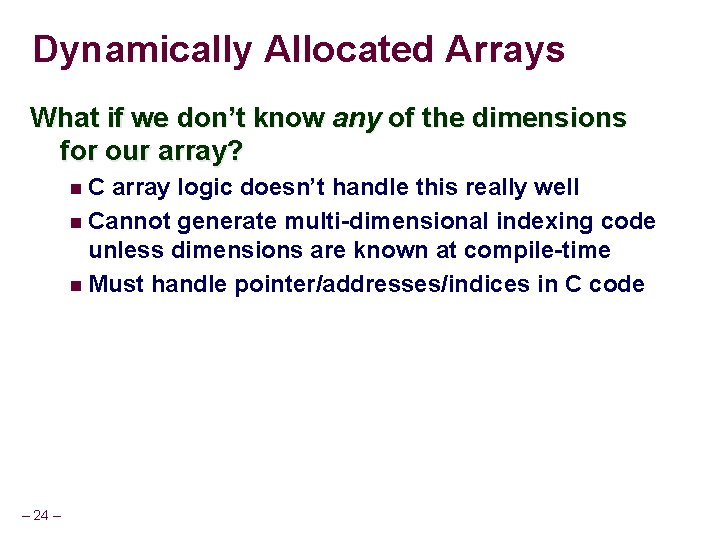Dynamically Allocated Arrays What if we don’t know any of the dimensions for our array? C array logic doesn’t handle this really well Cannot generate multi-dimensional indexing code unless dimensions are known at compile-time Must handle pointer/addresses/indices in C code – 24 –